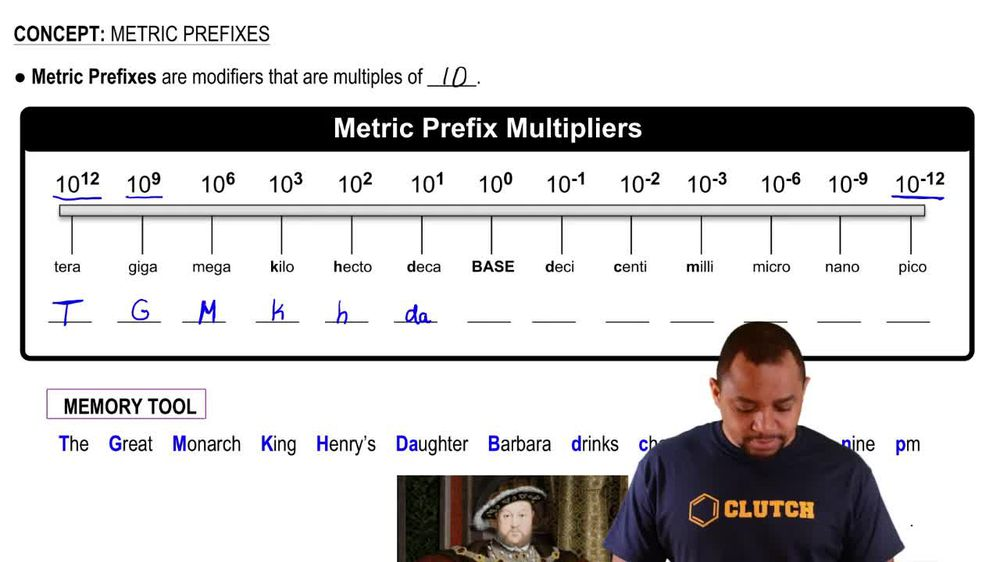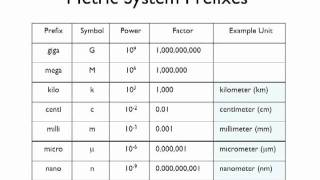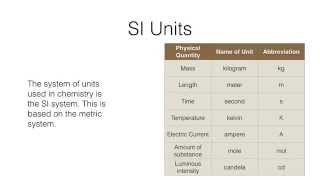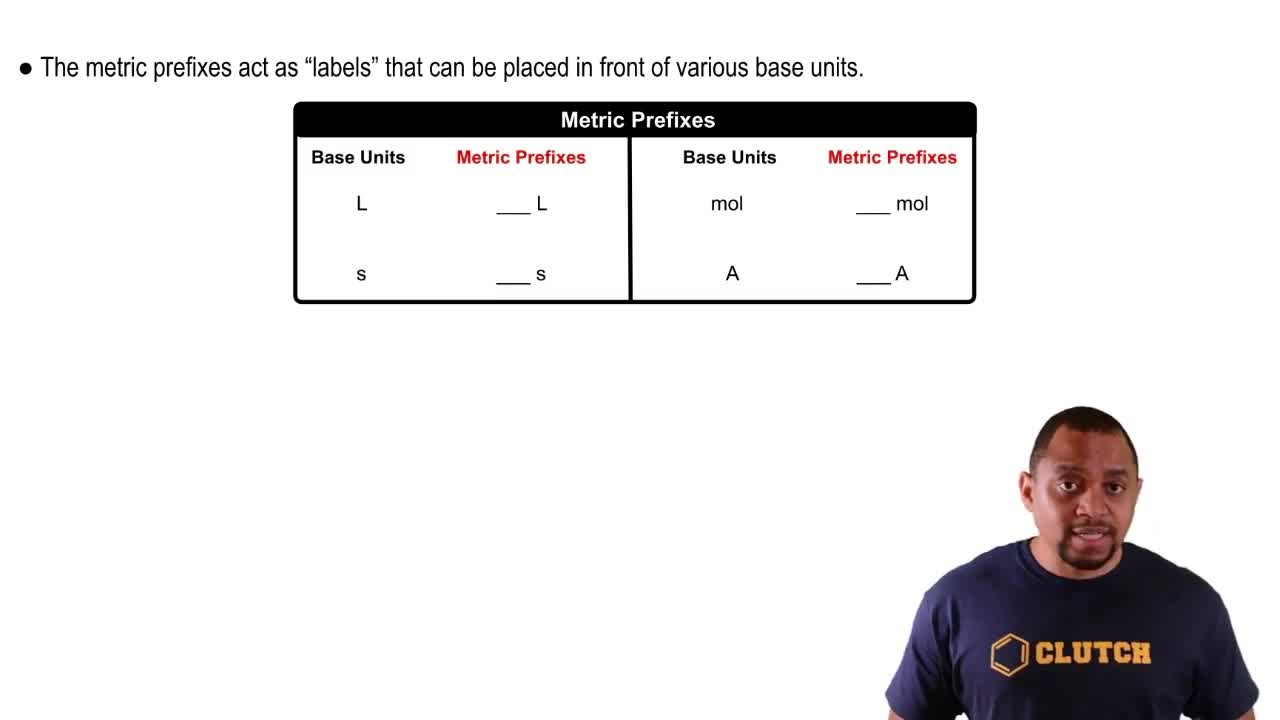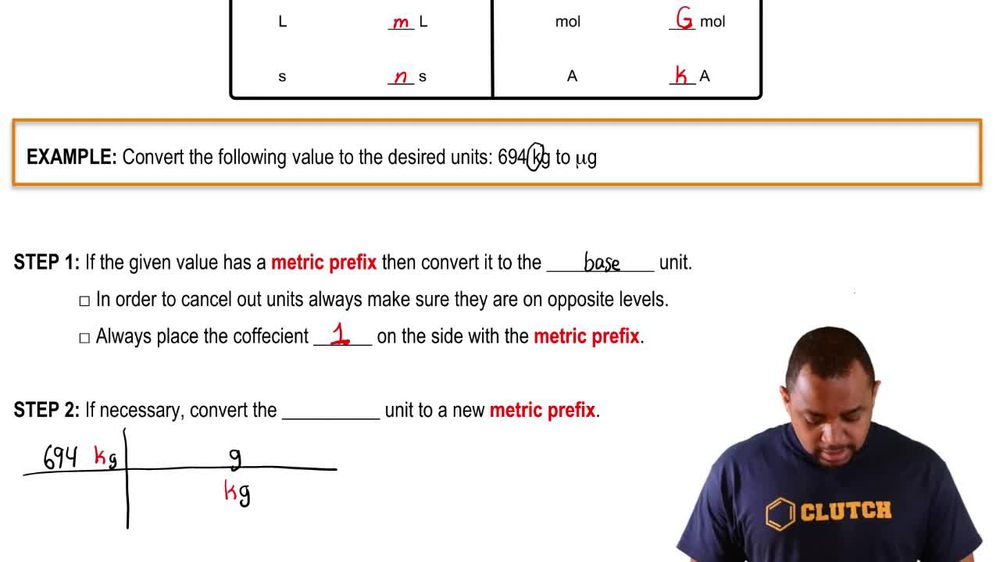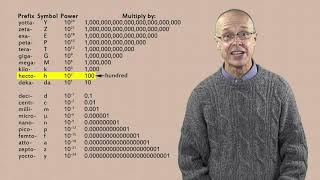Start typing, then use the up and down arrows to select an option from the list.
1. 1. Intro to General Chemistry2. Metric Prefixes
Problem

# Complete the following equivalent expressions by filling in the blanks. (a) 1 km = ____ m (b) 1m = _____ km (c) 1 mmol = _____ mol (d) 1 mol = _____ mmol

Relevant Solution1m
Play a video:
Hello everyone today we have a falling question completely equivalent expressions below by providing the missing values. So for a we have one in mole equals blank mole in is going to stand for nano and dana is going to be equivalent to 10 to the negative ninth moles. So we're gonna go ahead and write 10 to the negative ninth moles for B. We have one mole is equal to blank and animals. And so this is going to be the opposite 10 to the ninth. Since animals are smaller, it's going to take more to comprise of something that's bigger or regular moles for seat, We have one TM equals blank M&T. Is going to stand for Tara and terra is representative of 10 to the 12th meters, so one tera meter is equal to 10 to the 12th meters, meters are smaller than terra meters. And so for D we have the opposite happening here, We have one m is equal to blank terra meters and that's going to be 10 to the negative 12. Since terra meters are bigger, it's going to take less to comprise or something that's smaller than tara meters. And with that we have our answers. I hope this helped. And until next time